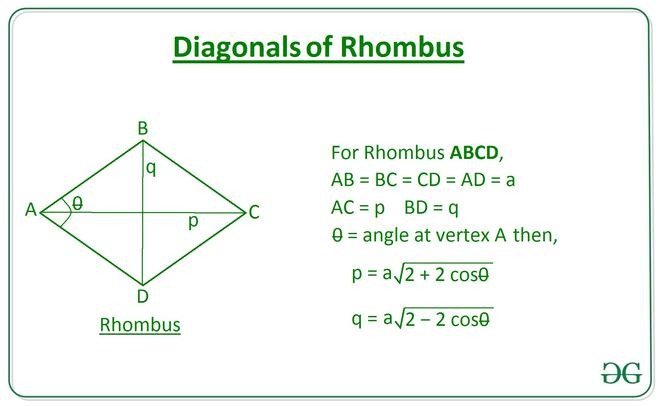# Length of diagonals of a Rhombus using length of Side and vertex Angle

• Last Updated : 11 May, 2021

Given two integers A and theta, denoting the length of a side of a rhombus and the vertex angle respectively, the task is to find the length of the diagonals of the rhombus.

Examples:

Input: A = 10, theta = 30
Output: 19.32 5.18

Input: A = 6, theta = 45
Output: 11.09 4.59

Approach:
The problem can be solved using the law of cosines. Using the law of cosines on triangles formed by the diagonals and sides of the rhombus gives the following relation to calculate the length of diagonals:Below is the implementation of the above approach:

## C++

 // C++ program to implement // the above approach #include  using namespace std;   // Function to calculate the length // of diagonals of a rhombus using // length of sides and vertex angle double Length_Diagonals(int a, double theta) {     double p = a * sqrt(2 + (2 * cos(            theta * (3.141 / 180))));     double q = a * sqrt(2 - (2 * cos(            theta * (3.141 / 180))));                  cout << fixed << setprecision(2) << p          << " " << q;  }   // Driver Code  int main()  {      int a = 6;     int theta = 45;         // Function Call      Length_Diagonals(a, theta);          return 0;  }    // This code is contributed by Virusbuddah_

## Java

 // Java program to implement // the above approach class GFG{   // Function to calculate the length // of diagonals of a rhombus using // length of sides and vertex angle static double[] Length_Diagonals(int a, double theta) {     double p = a * Math.sqrt(2 + (2 *                    Math.cos(theta * (Math.PI / 180))));       double q = a * Math.sqrt(2 - (2 *                    Math.cos(theta * (Math.PI / 180))));       return new double[]{ p, q }; }   // Driver Code public static void main(String[] args)  {     int A = 6;     double theta = 45;           double[] ans = Length_Diagonals(A, theta);       System.out.printf("%.2f" + " " + "%.2f",                       ans, ans); } }   // This code is contributed by Princi Singh

## Python3

 # Python Program to implement # the above approach import math   # Function to calculate the length # of diagonals of a rhombus using # length of sides and vertex angle def Length_Diagonals(a, theta):       p = a * math.sqrt(2 + (2 * \             math.cos(math.radians(theta))))                   q = a * math.sqrt(2 - (2 * \             math.cos(math.radians(theta))))       return [p, q]     # Driver Code A = 6 theta = 45   ans = Length_Diagonals(A, theta)   print(round(ans, 2), round(ans, 2))

## C#

 // C# program to implement // the above approach using System; class GFG{   // Function to calculate the length // of diagonals of a rhombus using // length of sides and vertex angle static double[] Length_Diagonals(int a, double theta) {     double p = a * Math.Sqrt(2 + (2 *                    Math.Cos(theta * (Math.PI / 180))));       double q = a * Math.Sqrt(2 - (2 *                    Math.Cos(theta * (Math.PI / 180))));       return new double[]{ p, q }; }   // Driver Code public static void Main(String[] args)  {     int A = 6;     double theta = 45;           double[] ans = Length_Diagonals(A, theta);       Console.Write("{0:F2}" + " " + "{1:F2}",                             ans, ans); } }   // This code is contributed by gauravrajput1

## Javascript

 

Output:

11.09 4.59

Time Complexity: O(1)
Auxiliary Space: O(1)

My Personal Notes arrow_drop_up
Recommended Articles
Page :